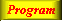Lecture 4 - Page 17 : 34
 Functional Programming in SchemeHigher-order Functions * Introduction to higher-order functions Higher-order functions Some simple and general higher-order functions Linear search in lists Generation of list selectors * Mapping and filtering Classical higher-order functions: Overview Mapping The mapping function Examples of mapping Filtering The filtering function Examples of filtering * Reduction and zipping Reduction The reduction functions Accumulation Zipping The zipping function * Currying The idea of currying Currying in Scheme Examples of currying Ad hoc currying in Scheme (1) Ad hoc currying in Scheme (2) * Web related higher-order functions HTML mirror generation HTML mirror usage examples Making tables with the real mirror Tables with higher-order functions HTML element modifications The function simple-html-table The XHTML mirror in LAML Generation of a leq predicate from enumeration
 Accumulation
 It is not satisfactory that we cannot reduce the empty listWe remedy the problem by passing an extra parameter to the reduction functionsWe call this variant of the reduction functions for accumulationThe function accumulate-right.

The recursive pattern is similar to the pattern of reduce-right.

 Expression Value `(accumulate-right - 0 '())` `0` `(accumulate-right - 0 '(1 2 3 4 5))` `3` ```(accumulate-right append '() (list (list 1 2 3) (list 'a 'b 'c)))``` `(1 2 3 a b c)`

Examples of right accumulations. The first row illustrates that we can accumulate the empty list. The second and third rows are similar to the second and third rows in Table 15.1.

 In relation to web programming we most often append accumulate lists and stringsaccumulate-right is part of the general LAML libraryDue to their deficiencies, the reduction functions are not used in LAML